# Rounding Numbers to Millions with Thousands as a Decimal in Microsoft Excel 2010

In this article we will learn about how we can round the numbers to millions with thousands as a decimal in Microsoft Excel.

To round the numbers in Millions with Thousands we can use the Custom Formator Round formula in Microsoft Excel 2010 and 2013.

We can round the numbers to Millions with thousands as a decimal from the 2 different ways.

1st Custom Format

2nd Round Function
Custom Format: -We can use Custom Number Format, to display a Number in Million or Display it in some Color.It’s a tool from in old versions of excel when there was no Conditional Formatting option in Excel. All conditional options are performed by using Custom Number Formal.
ROUND: - This function is used to round a number to a specified number of digits.

Syntax of “ROUND” function:       =ROUND (number, num_digits)

Example:Cell A1 contains the number 456.25

=ROUND (A1, 0), function will return 456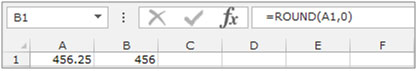Round Numbers to Millions with Thousands as a Decimal by using the Custom Format

Let’s take an example and understand.

We have categorized Income data. In which column D contains City Name, column E contains Gender and column F contains Income in \$. We want to format income in Millions.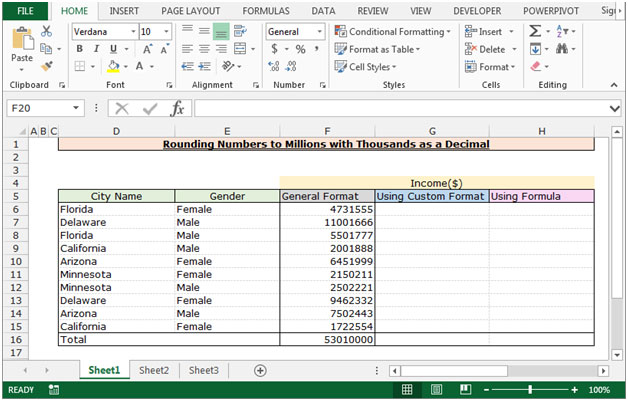To Round the Numbers to Millions with Thousands as a Decimal follow below given steps:-

• Select the range F6:F16 and copy by pressing the key Ctrl+C and paste in the range G6:G16 by pressing the key Ctrl+V on your keyboard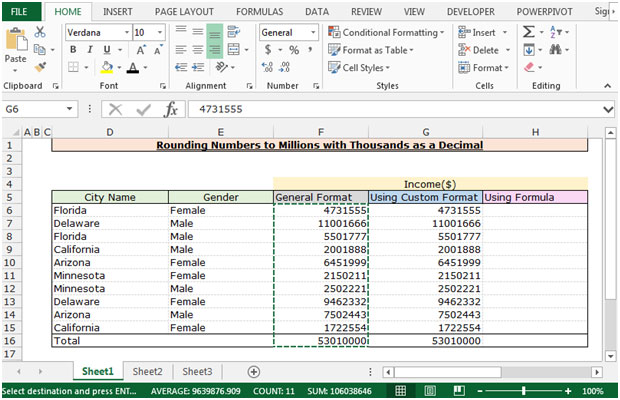• Select the range G6:G16 and press the key Ctrl+1 on your keyboard.
• Format Cells dialog box will appear.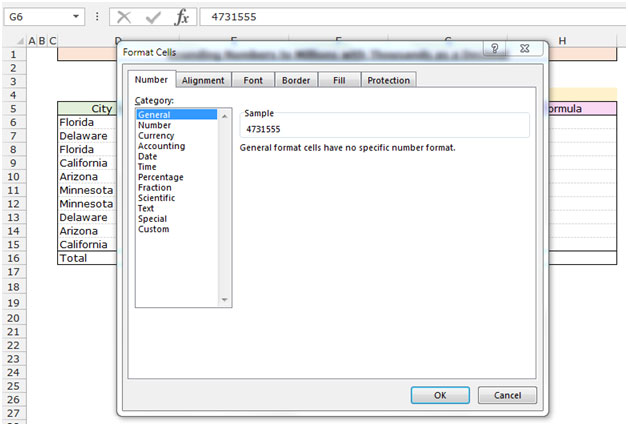• In the Number Tab, Click on Custom.
• After clicking on the Custom, related options will get appear.
• In the type box write the format type\$#.#,," M"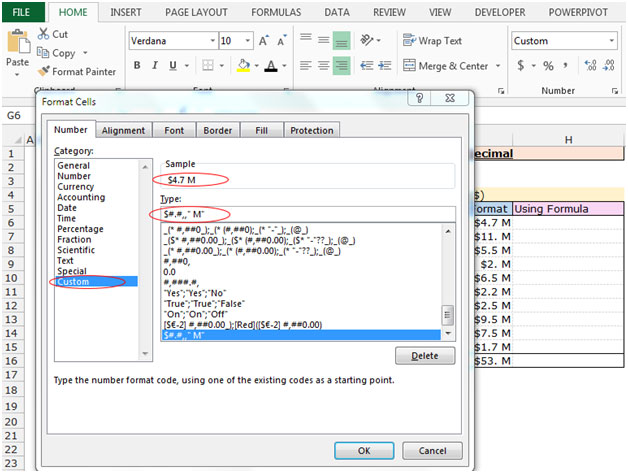• The function will return the rounded numbers to Millions with thousands as a decimal figures.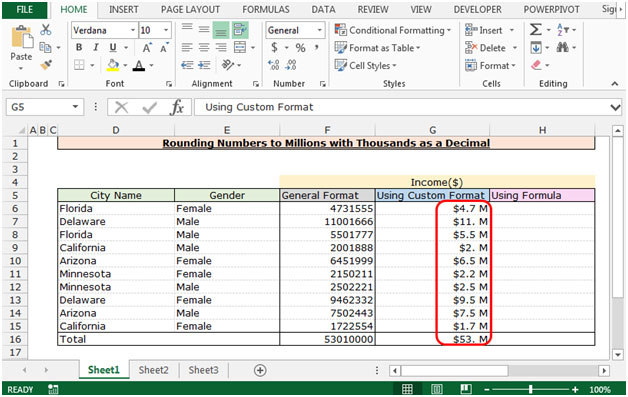Round the number by using the Round Function

Let’s understand with a simple exercise

• Select the Cell H6 and write the formula.
• ="\$"&ROUND((F6/1000000),1)&" M"press enter.
• The function will round the numbers to Millions with thousands as a decimal figures.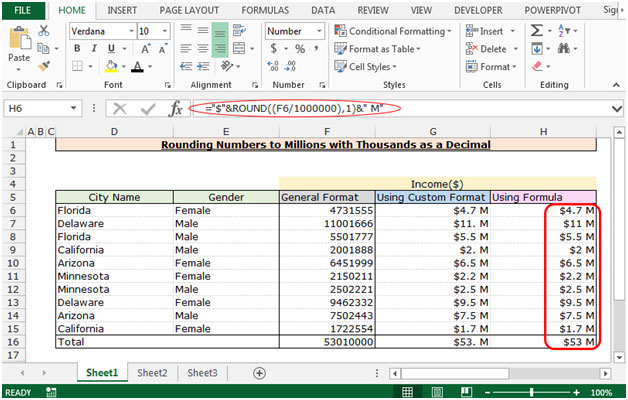This is the way we can round the numberto Million with Thousands as a decimal by using the formula and Custom Format in Microsoft Excel.

1.="\$"&Text(a1,"###,")&"K"
Also works if everything is going to be in the thousands...

2.0.0,, Works just as well

0.0 says that it must have 1 decimal
,, means that it must be divided by 1000 and another 1000 (each "," means divide by a thousand)

If you want to place text afterwards as Unit of measure use this:

0.0,, "MM"

This way is clearly noted that you are talking about Millions

3.Hi Ron. My only idea is the formula =LEN(text) which counts the characters for every cell. Maybe you have to add each cell. For example =(LEN(text)+LEN(TEXT)..this is just a one time set-up.

4."I'm trying to use a ""countif"" function in a 3 dimensional environment on a workbook that has pages representing months of the year.

I would like Excel to count all of the entries in Cell A1 on all of the pages, if there is anything there in any of them.

On a totals page, in Cell A1, I tried the following formula:

countif(Jan:Dec!A1,"">=a"")

It gives me a #Value error.

If I use:

countif(Dec!A1,"">=a"")

it works fine. So there must be something in the way I am defining the January to December worksheet pages that is messing up the formula, but I can't figure it out. Can you help me? "

5."Hello,

Is there an easier way to count the amount of text cells in a column automatically via a formula.

I understand I can put a 1 in a column next to each text cell in the previous column, then formulate to count the 1's, but I think thats extra work.

Isnt there a easier way to just add the amount of cells in a column that show text, as opposed to numbers, at the end of the column?

I dont/cant figure out if the COUNT function is able to do this or not, it appears to only work with numbers.

6.